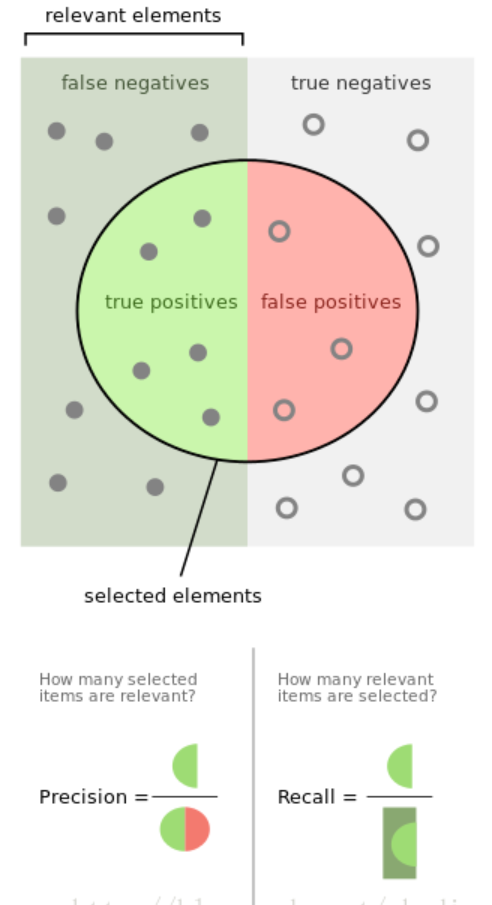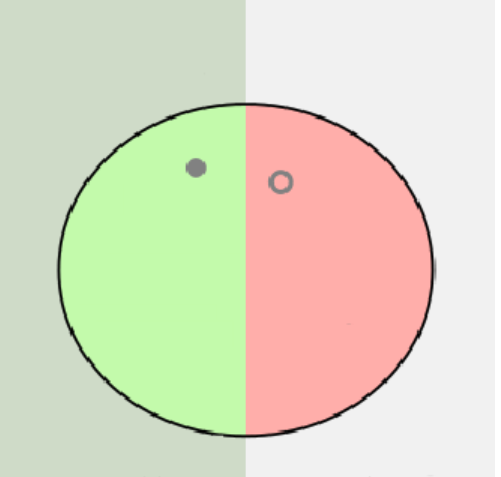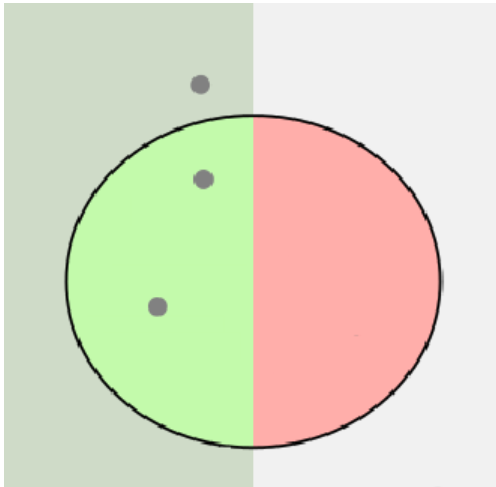机器学习classification_report方法及precision精确率和recall召回率 说明

classification_report简介

sklearn中的classification_report函数用于显示主要分类指标的文本报告．在报告中显示每个类的精确度，召回率，F1值等信息。

y_true：1维数组，或标签指示器数组/稀疏矩阵，目标值。
y_pred：1维数组，或标签指示器数组/稀疏矩阵，分类器返回的估计值。
labels：array，shape = [n_labels]，报表中包含的标签索引的可选列表。
target_names：字符串列表，与标签匹配的可选显示名称（相同顺序）。
sample_weight：类似于shape = [n_samples]的数组，可选项，样本权重。
digits：int，输出浮点值的位数．

classification_report用法示例：

from sklearn.metrics import classification_report
y_true = [0, 1, 2, 2, 2]
y_pred = [0, 0, 2, 2, 1]
target_names = ['class 0', 'class 1', 'class 2']
print(classification_report(y_true, y_pred, target_names=target_names))

precision    recall  f1-score   support

class 0       0.50      1.00      0.67         1
class 1       0.00      0.00      0.00         1
class 2       1.00      0.67      0.80         3

avg / total       0.70      0.60      0.61         5

precision recall f1-score三列分别为各个类别的精确度/召回率及 F1 F1值．

精确度/召回率/F1值

精确度＆召回率F1 F1值

F1 F1值是精确度和召回率的调和平均值：

2F1=1P+1R 2F1=1P+1R

F1=2P×RP+R F1=2P×RP+R

示例说明：

from sklearn.metrics import classification_report
y_true = [0, 1, 2, 2, 2]
y_pred = [0, 0, 2, 2, 1]
print(classification_report(y_true, y_pred))

precision    recall  f1-score   support

0       0.50      1.00      0.67         1
1       0.00      0.00      0.00         1
2       1.00      0.67      0.80         3

avg / total       0.70      0.60      0.61         5

0 0
1 0
2 2
2 2
2 1

precision    recall  f1-score   support

0       0.50      1.00      0.67         1
1       0.00      0.00      0.00         1
2       1.00      0.67      0.80         3P=12=0.5 P=12=0.5
R=11=1 R=11=1
F1=212×112+1=0.67 F1=212×112+1=0.67P=01=0 P=01=0
R=01=0 R=01=0
F1=0 F1=0P=22=1 P=22=1
R=23=0.67 R=23=0.67
F1=21×231+23+=0.8

posted @ 2018-03-13 16:34  178mz  阅读(20127)  评论(0编辑  收藏  举报